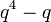# Linear representation theory of maximal unipotent subgroup of symplectic group of degree six over a finite field

## Contents

This article gives specific information, namely, linear representation theory, about a family of groups, namely: maximal unipotent subgroup of symplectic group of degree six. This article restricts attention to the case where the underlying ring is a finite field.
View linear representation theory of group families | View other specific information about maximal unipotent subgroup of symplectic group of degree six | View other specific information about group families for rings of the type finite field

## Summary

Item Value
number of conjugacy classes (equals number of irreducible representations over a splitting field) Case$q$ even (i.e., a power of 2): PLACEHOLDER FOR INFORMATION TO BE FILLED IN: [SHOW MORE]
Case$q$ odd:$q^4 + 4q^3 - 2q^2 - 3q + 1$
See number of irreducible representations equals number of conjugacy classes, element structure of maximal unipotent subgroup of symplectic group of degree six over a finite field
degrees of irreducible representations over a splitting field (such as$\overline{\mathbb{Q}}$ or$\mathbb{C}$) Case$q$ even (i.e., a power of 2): PLACEHOLDER FOR INFORMATION TO BE FILLED IN: [SHOW MORE]
Case$q$ odd: 1 (occurs$q^3$ times),$q$ (occurs$q^4 - q$ times),$q^2$ (occurs$2q^3 - 2q^2$ times),$q^3$ (occurs$q^3 - 2q + 1$ times)
sum of squares of degrees of irreducible representations$q^9$ (equals order of the group)
see sum of squares of degrees of irreducible representations equals order of group
lcm of degrees of irreducible representations$q^3$
condition for a field (characteristic not equal to$p$) to be a splitting field PLACEHOLDER FOR INFORMATION TO BE FILLED IN: [SHOW MORE]
field generated by character values, which in this case also coincides with the unique minimal splitting field (characteristic zero) PLACEHOLDER FOR INFORMATION TO BE FILLED IN: [SHOW MORE]
unique minimal splitting field (characteristic$c \ne 0,p$) PLACEHOLDER FOR INFORMATION TO BE FILLED IN: [SHOW MORE]